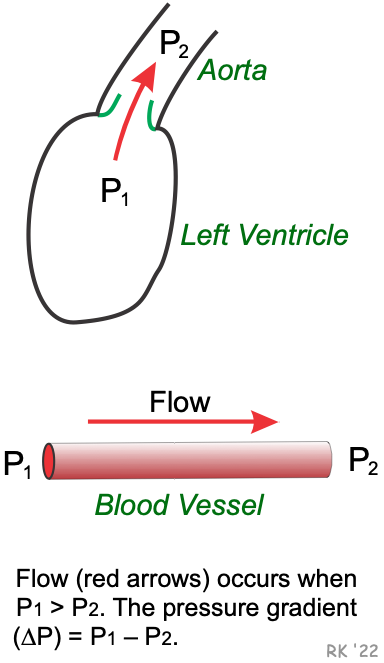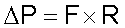In order for blood to flow through a vessel or across a heart valve, there must be a force propelling the blood. This force is the difference in blood pressure (i.e., pressure gradient) across the vessel length or across the valve (P- P2 in the figure). At any pressure gradient (ΔP), the flow rate is determined by the resistance (R) to that flow. The factors determining the resistance are described by the Poiseuille relationship. The most important factor, quantitatively and functionally, is the radius of the vessel, or, with a heart valve, the orifice area of the opened valve. Resistance is inversely related to the fourth power of the radius (r4) of a blood vessel. For heart valves, it is not possible to use an orifice radius because the opening is not circular. Therefore, the area of the valve orifice is used to compute resistance instead of radius, where area (A) is proportional to the square of the radius (r2), based upon the equation A = π r2. For a heart valve, therefore, the resistance to flow is inversely proportional to A2.

The pressure gradient can be viewed as the force driving flow (F), where F = ΔP/R. This relationship is based upon Ohm's Law from physics in which current (I) equals the voltage difference (ΔV) divided by the resistance (R) (i.e., I= ΔV/R). Flow is decreased, for example, if there is a decrease in ΔP or an increase in R, as shown in the figure below. In this example, ΔP is an independent variable, whereas flow is the dependent variable.

The pressure gradient can also be viewed as the pressure drop (i.e., energy loss) that results at a given flow and resistance (i.e., ΔP is the dependent variable), where ΔP=F x R. In summary, ΔP is increased by either an increase in flow or resistance.For example, under laminar flow conditions, doubling the flow across a heart valve or along a length of blood vessel doubles the pressure drop across the valve or along the vessel length.

A normal valve, like a normal large artery, has a very small resistance to flow, and therefore, the pressure gradient across the valve is very small. In contrast, with vascular or valvular stenosis (narrowing) the pressure gradient is increased because of the increased resistance to flow (e.g., by decreased vessel radius or valve cross-sectional area). As flow increases across the stenotic lesion (e.g., when cardiac output increases during exercise), the pressure gradient (ΔP) increases further. Increased flow across a heart valve, particularly when it is stenotic, causes a large increase in velocity that can lead to a significant turbulence, which will further augment the pressure gradient across the valve and lead to a functional murmur or enhance the intensity of a preexisting murmur.

Revised 12/27/2022

DISCLAIMER: These materials are for educational purposes only, and are not a source of medical decision-making advice.